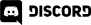# Home > Programming >

18. November 2012

This recipe looks at how to rotate one sprite relative to another point.  In this example, we rotate a jet sprite to face the position of the mouse.

Mouse over the application to your right to see how the centred sprite follows the mouse cursor.  You may need to tap the screen to focus the mouse. As you move the mouse you can see the angle between the sprite and the mouse cursor.  The X and Y values represent the current location of the mouse on the HTML canvas.  You may notice that 0/360 degrees is to right hand side, this is a side effect of the Atan2 method.

## Just The Math

var angle = Math.atan2(stage.mouseY - jetSprite.y, stage.mouseX - jetSprite.x );

angle = angle * (180/Math.PI);

## Description

First we get the distance between the sprite and the mouse.  This is obtained simply by subtracting the mouse location from the sprite location.  We then take the atan2 of the resulting coordinates.  One warning with atan2, the parameters are (y,x) not (x,y)!  Atan2 will return the angle, with a few gotchas.  First off, the angle is returned in radians.  Depending on your graphic library, you may need to convert to degrees to perform a rotation ( such as the case with EaselJs ).  If your language/library of choice doesn't have a RadiansToDegrees function, the formula is simply degrees = radiansToConvert * (180 divided by Pi).  Keep in mind, Pi (π 3.14159____ ) represents half a circle, so 2π is a complete circle( 360 degrees ), 1/2π is 90 degrees, etc.

Second, the angle is relative to the positive X axis, meaning that 0 degrees is pointing right.  If you look at the full source code to the example, you will notice that the source image itself actually points up, so we increase the angle by 90 degrees to adjust for orientation of the jet sprite image.  Also, depending on origin your graphic library uses, you may need to flip the sign in the y direction.

One other side effect of atan2 is it returns the results as a value range of -180 to 180.  If you want the value to be 0 to 360, perform the following:

if(angle < 0)

{

angle = 360 - (-angle);

}

## The Complete Code

<!DOCTYPE html>

<html>

<script src="http://code.createjs.com/easeljs-0.5.0.min.js"></script>

<script>

var jetSprite;

var stage;

var textOut;

function demo(){

stage = new createjs.Stage("theCanvas");

// onFrame will be called each "tick". Default is 50ms, or 20FPS

// Create and configure our jet sprite. regX/Y set the pivot point, we center it

jetSprite = new createjs.Bitmap("jetsprite.png");

jetSprite.regX = jetSprite.image.width/2;

jetSprite.regY = jetSprite.image.height/2;

jetSprite.x = stage.canvas.width/2;

jetSprite.y = stage.canvas.height/2;

//Now we create a Text object, used for displaying some debug details

textOut = new createjs.Text("Debug","24px Arial","red");

textOut.x = 5;

textOut.y = 5;

textOut.maxWidth = 390;

//All the worlds a stage after all... add our sprite and text to it

//And go...

stage.update();

}

function onFrame(elapsedTime) {

var angle = Math.atan2(stage.mouseY - jetSprite.y, stage.mouseX - jetSprite.x );

angle = angle * (180/Math.PI);

// The following if statement is optional and converts our angle from being

// -180 to +180 degrees to 0-360 degrees.  It is completely optional

if(angle < 0)

{

angle = 360 - (-angle);

}

textOut.text = "X:" + stage.mouseX + " Y:" + stage.mouseY + " angle:" + Math.round(angle);

// Atan2 results have 0 degrees point down the positive X axis, while our image is pointed up.

// Therefore we simply add 90 degrees to the rotation to orient our image

// If 0 degrees is to the right on your image, you do not need to add 90

jetSprite.rotation =90 + angle;

stage.update();

}

</script>

<canvaswidth=400pxheight=400pxid="theCanvas"style="background-color:black"/>

</body>

</html>

http://gamedev.stackexchange.com/questions/14602/what-are-atan-and-atan2-used-for-in-games

The results of Atan2 can be a bit confusing, depending on the way your brain works.  In the full source code, you can see that I add 90 degrees to the rotation angle.  This is because the image ( and my mindset ) view 0 degrees as being up the positive Y axis.  Of course, this is completely arbitrary.

Consider for a moment the philosophical question "where does a circle begin?".  The answer completely depends on who you ask.  If you ask me, 0 degrees is up.  If you ask atan2, 0 degrees is to the right.  Consider the following diagram:As you can see, the position atan2 considers to be zero is offset 90 degrees from the position I consider ( and have drawn my art according to ) to be 0 degrees.  Fortunately the answer is as simple as adding 90 degrees to the angle during rotations.  Of course, you could simply draw your art as if 0 degrees was along the positive X axis and you won't have any problems.